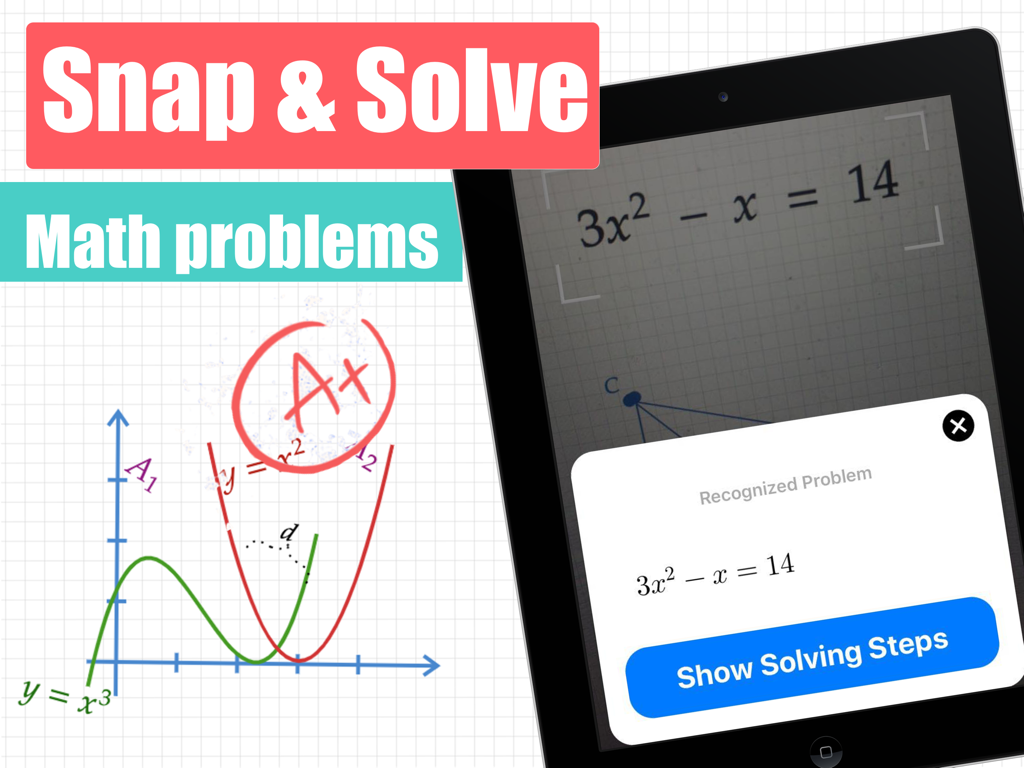# Math Box – Math Answer Scanner – Quantty LTDMath Box – Math Answer Scanner Quantty LTD Genre: Education Release Date: January 3, 2020

MathBox does the math homework for you. Just snap a photo of any math problem, and voila – you get clear step by step solution explanation. Get unstuck in math and learn maths better. MathBox will help you solve your math homework faster and have better preparation for math classes and exams. MathBox will provide you with a clear step by step explanation of every math problem you have at school.

MathBox App Features:

● Take a photo of any math problem.
● The app Calculates and Solves the math problem.
● MathBox handles Handwriting math Problems.
● Step-by-step Solution with clear explanation.
● Graphical and function plots for a perfect explanation.
● Save your results in the "History" tab so you can go back to them anytime.
● Save your results in PDF and share them with friends easily.

MathBox covers many math topics for any age or grades:

● Basic math: pre-algebra, integers, rational numbers, decimal numbers, additions, multiplications, fractions, powers, roots, factors, simplifications, exponents, …
● Algebra: polynomial equations, linear equations with one variable, linear equations with many variables, linear inequalities, quadratic equations, systems of equations, logarithms, graphs, …
● Calculus: functions graphs, limits, integrals, derivatives, …
● And many more topics

Math could be hard sometimes – but with MathBox , it’s never been simpler and easier :).